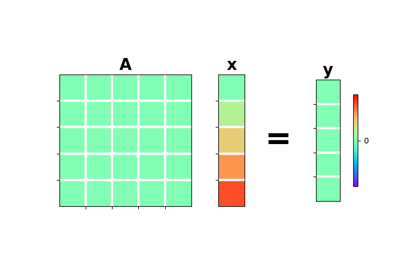# pylops.Zero¶

class pylops.Zero(N, M=None, dtype='float64')[source]

Zero operator.

Transform model into array of zeros of size $$N$$ in forward and transform data into array of zeros of size $$N$$ in adjoint.

Parameters: N : int Number of samples in data (and model in M is not provided). M : int, optional Number of samples in model. dtype : str, optional Type of elements in input array.

Notes

An Zero operator simply creates a null data vector $$\mathbf{y}$$ in forward mode:

$\mathbf{0} \mathbf{x} = \mathbf{0}_N$

and a null model vector $$\mathbf{x}$$ in forward mode:

$\mathbf{0} \mathbf{y} = \mathbf{0}_M$
Attributes: shape : tuple Operator shape explicit : bool Operator contains a matrix that can be solved explicitly (True) or not (False)

Methods

 __init__(self, N[, M, dtype]) Initialize this LinearOperator. adjoint(self) Hermitian adjoint. cond(self, \*\*kwargs_eig) Condition number of linear operator. conj(self) Complex conjugate operator div(self, y[, niter]) Solve the linear problem $$\mathbf{y}=\mathbf{A}\mathbf{x}$$. dot(self, x) Matrix-matrix or matrix-vector multiplication. eigs(self[, neigs, symmetric, niter]) Most significant eigenvalues of linear operator. matmat(self, X) Matrix-matrix multiplication. matvec(self, x) Matrix-vector multiplication. rmatvec(self, x) Adjoint matrix-vector multiplication. transpose(self) Transpose this linear operator.

## Examples using pylops.Zero¶Zero